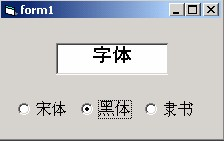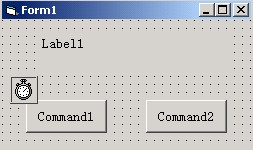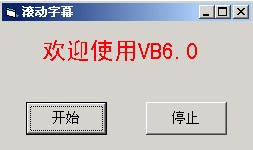﻿ 往年试卷-青岛科技大学 - 信息科学技术学院

1 ．刚建立一个新的标准 EXE 工程后，不在工具箱中出现的控件是

A. 图片框 B. 单选按钮 C. 通用对话框 D. 文本框

2 ．工程文件的扩展名是

A. .frm B. .vbp C. .bas D. .res

3 ．在设计阶段，当双击窗体上的某个控件时，所打开的窗口是

A. 工程资源管理器窗口 B. 工具箱窗口 C. 代码窗口 D. 属性窗口

4 ．当一个工程含有多个窗体时，默认的启动窗体是

A. 启动 VB 时建立的窗体 B. 第一个添加的窗体

C. 最后一个添加的窗体 D. 在工程属性对话框中指定的窗体

5 ．以下变量名不正确的是

A.myname B. 学校 \$ C.ab-2 D.Print_3

6 ．确定一个控件在窗体上的位置的属性是

A.Width 和 Height B.Width 或 Height C.Top 和 Left D.Top 或 Left

7 ．设 a=2,b=3,c=4,d=5 ，表达式： Not a<=c Or 4*c=b^2 And b<>a+c 的值是

A. -1 B. 1 C. True D. False

8 ．以下赋值语句错误的是

A.a=a+1 B.a=4>3 C.a=true+2 D.a="true"+2

9 ．执行语句 s=Len(Mid(“VisualBasic”,1,6)) 后， s 的值是

A. Visual B. Basic C.6 D.11

10 ．下面程序段求两个数中的大数，不正确的是

A.max=IIf(x>y ， x ， y) B. If x>y then max=x Else max=y

C.max=x ： If y>=x Then max=y D. If y>=x then max=y ： max=x

11 ．区分控件数组中不同元素的属性是

A ． Name B ． Index C ． Caption D ． Height

12 ．图像框有一个属性，可以自动调整图形的大小，以适应图像框的尺寸，这个属性是

A. Autosize B. Stretch C. AutoRedraw D. Appearance

13 ．以下能够触发文本框 Change 事件的操作是

A. 文本框失去焦点 B. 文本框获得焦点 C. 设置文本框的焦点 D. 改变文本框的内容

14 ． Dim b(2 To 5,-2 to 3) 所定义的数组的元素的个数是

A.20 B.12 C.15 D.24

15 ．默认情况下，用 Inputbox 函数返回的数据类型是

A.Integer B.String C.Single D.Byte

16 ．可以作为其他控件的容器的是

A.Form B.CommandButton C.Label D.Image

17 ．以下叙述中错误的是

A. 一个工程中只能有一个 Sub Main 过程

B. 窗体的 Show 方法的作用是将指定的窗体装入内存并显示该窗体

C. 窗体的 Hide 方法和 Unload 方法的作用完全相同

D. 若工程文件中有多个窗体，可以根据需要指定一个窗体为启动窗体

18 ．删除列表框或组合框中指定的项目所使用的方法是

A.Move B.RemoveItem C.Clear D.SetFocus

19 ．设 a= “ Visual Basic ”，下面使 b= “ Basic ”的语句是

A.b=Left(a,8,12) B.b=Mid(a,8,5) C.b=Right(a,5,5) D.b= Left(a,8,5)

20 ．以下关系表达式中 , 其值为 False 的是

A.”ABC”>”Abc” B.”the”>”they” C.”VISUAL”=UCase(“Visual”) D.”Interger”>”Int”

21 ．下列控件中，没有 Captoin 属性的是

A. 框架 B. 列表框 C. 复选框 D. 单选按钮

22 ．设在菜单编辑器中定义了一个菜单项，名为 mnu1 ，为了在运行时隐藏该菜单项，应使用的语句是

A ． mnu1.Enabled= Flase B ． mnu1.Enabled= True

C ． mnu1.Visible= Flase D ． mnu1.Visible= True

23 ． Int(Rnd*100) 返回的整数范围是

A. （ 1 ， 99 ） B. （ 1 ， 100 ） C. [0 ， 100] D. [0 ， 99]

24 ．下列鼠标事件中不能区分出按了鼠标哪一个键的是

A. Click B. MouseMove C. MouseDown D. MouseUp

25 ．可以判断在鼠标事件中是否按了 Alt 键的参数是

A.Button B.Shift C.X D.Y

26 ．以下 Case 语句中错误的是

A. Case 0 to 10 B. Case Is >10 C. Case Is >10 And Is <50 D. Case 3,5,Is >10

27 ．能返回组合框中选中的数据项索引值的是

A.ListCount B.ListIndex C.Text D.List

28 ．关于过程嵌套，说法正确的是

A. 过程定义和过程调用都不可嵌套 C. 过程定义可嵌套，过程调用不可嵌套

B. 过程定义和过程调用都可嵌套 D. 过程定义不可嵌套，过程调用可嵌套

29 ．既能定义模块级变量又能定义过程级变量的是

A.Dim B.Public C.Private D.Static

30 ．复选框的 Value 属性为 0 时，表示的是

A．复选框未被选中 B．复选框被选中但呈灰色

C．复选框被选中 D．数据类型不匹配

1．结构化程序的三种基本结构是 _______ 、 _______ 、 _______ 。

2．定时器事件之间的间隔通过 _______ 属性设置。

3．要添加一幅画作为窗体的背景，应设置窗体的 _______ 属性。

4．运行程序时，欲使某一个控件首先得到焦点，可在代码中对该控件使用 _______ 方法。

5．希望窗体先于控件接收键盘事件，需要修改窗体的 _______ 属性。

6．将相同类型的一组控件的 _______ 属性设为相同，则产生控件数组。

7．当拖动滚动条中的滚动块时，将触发滚动条的 _______ 事件。

8． _______ 方法可清除列表框或组合框中的所有内容。

1．Private Sub Text1_KeyPress(KeyAscii As Integer)
KeyAscii=Asc(LCase(Chr(KeyAscii)))
End Sub

2．Private Sub Form _click( )
x=1:y=2
Do while y<=4
x=x*y:y=y+1
Loop
Print x
End Sub

3．Option base 1
Private Sub Form_Click( )
Dim a, i as Integer, j as Integer, s as Integer
a=Array(1,2,3)
j=1
For i=1 to 3
s=s+a(i)*j
j=j*2
Next i
Print s
End Sub

4．Private Sub Form_Click( )
Dim a(4,3) as Integer
Print Ubound(a,2)
End Sub

5．Private Sub mysub(a as Integer, b as Integer, c as Integer )
a=a*2: b=b*3: c=c*4
End Sub
Private Sub Form_Click( )
Dim x as Integer, y as Integer
x=2: y=3
Call mysub(x+y, y+1, x)
Print x, y
End Sub

1．以下程序是求“ 1 ！ +2 ！ +3 ！ +…+n! ”。请将程序补充完整。
Private Sub Command1_click( )
Dim i As Integer ， n As Integer ， jc As Long ， s As Long
n =InputBox （“请输入 n 的值：”）
jc=1
For i=1 to n
________
s=s+jc
Next i
Print “1!+2!+3!+ ··· +”;n; “!=”; s
End Sub

2．以下程序是比较两个数的大小。请将程序补充完整。
Private Sub Form_Click( )
Dim a% ， b% ， c%
a=InputBox （“请输入 a 的值：”）
b=InputBox （“请输入 b 的值：”）
If a<b then c=a ： ________ ： b=c
Print a ；“ > ”； b
End Sub

3．在窗体上画一个名称为 Text1 的文本框，然后画三个单选按钮，并用这三个单选按钮建立一个控件数组，名称为 Option1 。程序运行后，如果单击某个单选按钮，则文本框中的字体将根据所选择的单选按钮切换，如图所示。请将程序补充完整Private Sub Option1_Click(Index As Integer)
Select Case ________
Case 0
a = " 宋体 "
Case 1
a = " 黑体 "
Case 2
a = " 隶书 "
End Select
Text1.________= a
End Sub

4．以下程序是利用“比较交换法”对 10 个数据按升序排列。请将程序补充完整。
Option Base 1
Private Sub Form_Click()
Dim a, i%, j%, t%, n%
a = Array(84, 92, 70, 37, 25, 75, 50, 65, 35,97)
n = UBound(a)
For i = 1 To n - 1
For j = i + 1 To n
If________ Then
t = a(i): a(i) = a(j): a(j) = t
End If
Next j
Print a(i);
Next i
Print a(n)
End Sub

5．以下程序是输出一个 5 × 5 的方阵，其中的元素是随机产生的 10~99 两位整数。请将程序补充完整。
Option Base 1
Dim a(5, 5) As Integer , i As Integer, j As Integer
Private Sub Form_Click()
Randomize
For i = 1 To 5
For j = 1 To 5
________
Print a(i, j);
Next j
Print
Next i
End Sub

1. 输出下列图形：
*********
*******
*****
***
*

2．求： 的前 n 项之和， n 由键盘输入。

3．滚动字幕设计。在窗体上添加两个命令按钮（名称分别为 command1 、 command2 ）、一个标签（名称为 label1 ）、一个定时器（名称为 timer1 ），设计界面如图所示。运行时，窗体标题为“滚动字幕”；标签显示为“欢迎使用 VB6.0 ”，黑体、 18 号字、红色，背景透明；两个命令按钮分别显示为“开始”、“停止”。单击“开始”按钮，标签每隔 1 秒钟向左边移动一次，当从左边离开窗体后，又从右边进入窗体，这一过程循环进行。单击“停止”按钮，标签停止移动。（要求：所有控件的非默认属性由代码设置）